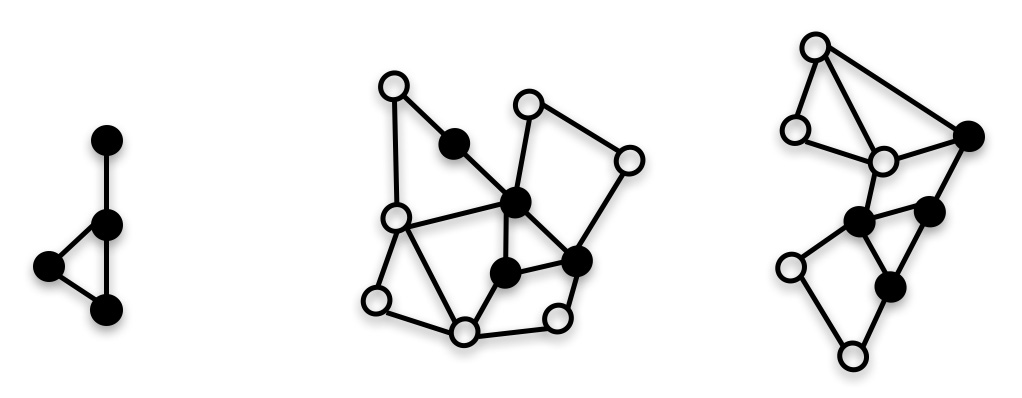Browse SoTA > Graphs > Graph Clustering# Graph Clustering Edit

37 papers with code · Graphs

Graph Clustering is the process of grouping the nodes of the graph into clusters, taking into account the edge structure of the graph in such a way that there are several edges within each cluster and very few between clusters. Graph Clustering intends to partition the nodes in the graph into disjoint groups.

# Variational Graph Auto-Encoders

21 Nov 2016tkipf/gaeWe introduce the variational graph auto-encoder (VGAE), a framework for unsupervised learning on graph-structured data based on the variational auto-encoder (VAE).

1,048

# A Non-negative Symmetric Encoder-Decoder Approach for Community Detection

Latent factor models for community detection aim to find a distributed and generally low-dimensional representation, or coding, that captures the structural regularity of network and reflects the community membership of nodes.

877

# Symmetric Nonnegative Matrix Factorization for Graph Clustering

Unlike NMF, however, SymNMF is based on a similarity measure between data points, and factorizes a symmetric matrix containing pairwise similarity values (not necessarily nonnegative).

877

# Optimal Transport for structured data with application on graphs

23 May 2018rflamary/POT

This work considers the problem of computing distances between structured objects such as undirected graphs, seen as probability distributions in a specific metric space.

729

# Cluster-GCN: An Efficient Algorithm for Training Deep and Large Graph Convolutional Networks

benedekrozemberczki/ClusterGCNFurthermore, Cluster-GCN allows us to train much deeper GCN without much time and memory overhead, which leads to improved prediction accuracy---using a 5-layer Cluster-GCN, we achieve state-of-the-art test F1 score 99. 36 on the PPI dataset, while the previous best result was 98. 71 by .

418

# Graph-Bert: Only Attention is Needed for Learning Graph Representations

15 Jan 2020jwzhanggy/Graph-BertWe have tested the effectiveness of GRAPH-BERT on several graph benchmark datasets.

126

# Adversarially Regularized Graph Autoencoder for Graph Embedding

13 Feb 2018Ruiqi-Hu/ARGAGraph embedding is an effective method to represent graph data in a low dimensional space for graph analytics.

120

# A Survey of Adversarial Learning on Graphs

10 Mar 2020gitgiter/Graph-Adversarial-LearningTo bridge this gap, we investigate and summarize the existing works on graph adversarial learning tasks systemically.

94

# Residual Gated Graph ConvNets

xbresson/spatial_graph_convnetsIn this paper, we are interested to design neural networks for graphs with variable length in order to solve learning problems such as vertex classification, graph classification, graph regression, and graph generative tasks.

91

# $p$-Norm Flow Diffusion for Local Graph Clustering

20 May 2020kfoynt/LocalGraphClustering

Local graph clustering and the closely related seed set expansion problem are primitives on graphs that are central to a wide range of analytic and learning tasks such as local clustering, community detection, nodes ranking and feature inference.

60